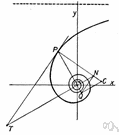# hyperbolic geometry

(redirected from Gauss-Bolyai-Lobachevsky space)
Also found in: Thesaurus, Encyclopedia.
Related to Gauss-Bolyai-Lobachevsky space: Lobachevskian Geometry
ThesaurusAntonymsRelated WordsSynonymsLegend:
 Noun 1hyperbolic geometry - (mathematics) a non-Euclidean geometry in which the parallel axiom is replaced by the assumption that through any point in a plane there are two or more lines that do not intersect a given line in the plane; "Karl Gauss pioneered hyperbolic geometry"math, mathematics, maths - a science (or group of related sciences) dealing with the logic of quantity and shape and arrangementnon-Euclidean geometry - (mathematics) geometry based on axioms different from Euclid's; "non-Euclidean geometries discard or replace one or more of the Euclidean axioms"
Site: Follow: Share:
Open / Close